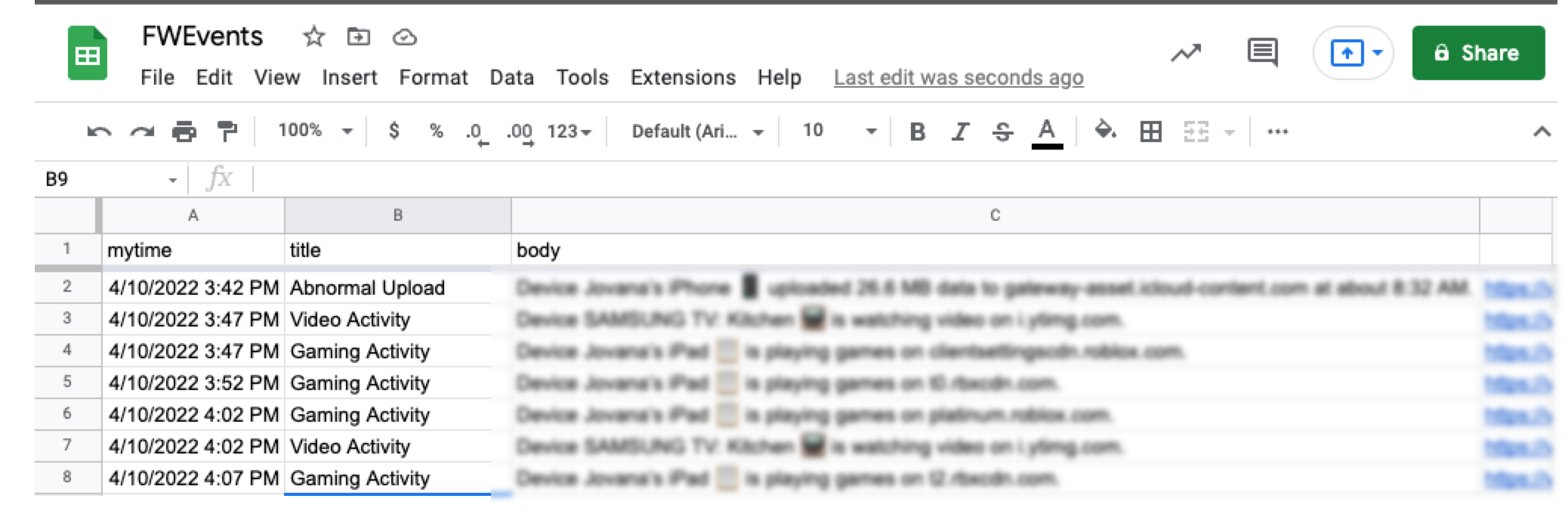Instantly share code, notes, and snippets.

# oscarmorrison/IFTTTDate.md

Last active May 22, 2023 14:04
Star You must be signed in to star a gist

##Date and Time

``````=TIMEVALUE(SUBSTITUTE("{{OccurredAt}}"," at ", " ")) + DATEVALUE(SUBSTITUTE("{{OccurredAt}}"," at ", " "))
``````

##Date

``````=DATEVALUE(SUBSTITUTE("{{OccurredAt}}"," at ", " "))
``````

##Time

``````=TIMEVALUE(SUBSTITUTE("{{OccurredAt}}"," at ", " "))
``````

### To Use

Simple just place either or both separated by ||| in the
Formatted Row Input on IFTTT### mbierman commented Apr 10, 2022 • edited

Uh... there is a much better way to deal with this.

In IFTTT, you add a filter you can format the date so you don't then have to play with it in the Sheet side.

For example, using the following filter:

``````let mytime = Meta.currentUserTime.format('l LT');
let update=MakerWebhooks.event.Value1;

``````

Which will format the all of the entries like so:### moomdate commented Nov 9, 2022

\m/

```function myFunction(e) {
Logger.log(JSON.stringify(e));
if(e && e.changeType === 'INSERT_ROW') {
var sheet = ss.getSheetByName("Sheet1");
sheet.getRange(sheet.getLastRow(),1).setValue(new Date());
}
}```

### OliverK-dev commented Dec 8, 2022 • edited

In Google Sheets you can just use this formula:

`=TIMEVALUE(SUBSTITUTE(A1," at ", " ")) + DATEVALUE(SUBSTITUTE(A1," at ", " "))`

I simply replaced {{OccurredAt}} with the cell reference -- i.e., A1